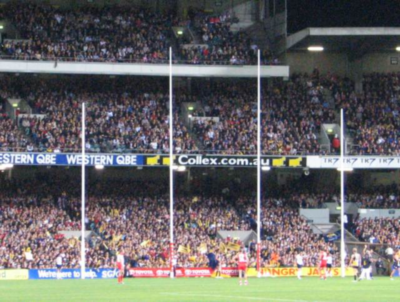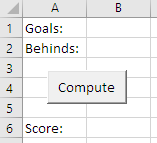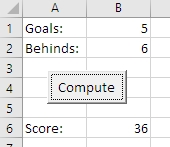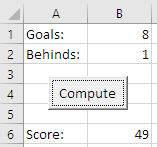# GeneralizeNoms?
Photo: Holly Vickery

### Two programs

Here are the two programs again. The tip program, and the feet to meters program.

• Dim sMealCost As Single
• Dim sTip As Single
• Dim sTotal As Single
• sMealCost = Cells(1, 2)
• sTip = sMealCost * 0.15
• sTotal = sMealCost + sTip
• Cells(5, 2) = sTip
• Cells(6, 2) = sTotal
•
•
• Dim sInchesInput As Single
• Dim sFeetInput As Single
• Dim sInchesTotal As Single
• Dim sMeters As Single
• sInchesInput = Cells(5, 2)
• sFeetInput = Cells(6, 2)
• sInchesTotal = sFeetInput * 12 + sInchesInput
• sMeters = sInchesTotal * 2.54
• Cells(10, 2) = sMeters

(I've left out the `Sub` lines and some other stuff, as well as the first level of indenting.)

Let's generalize. That is, see what the programs have in common, so we can work out a pattern for this type of program.

What do the programs have in common?

### An input patternEthan

It looks like they get input from cells into variables.

Aye, they do.

• sMealCost = Cells(1, 2)
•
• sInchesInput = Cells(5, 2)
• sFeetInput = Cells(6, 2)

In each line, there's a cell reference on the right of the =. Data comes out of the cell, and into the variable on the left of the =.Ray

Is all input like that?

No, we'll see another way of doing it soon. But a lot of input is like that.

It's so common, let's make a pattern out of it. A pattern in a common way of doing things.

Pattern

Get input from a cell.

Anything else the two programs have in common?

• Dim sMealCost As Single
• Dim sTip As Single
• Dim sTotal As Single
• sMealCost = Cells(1, 2)
• sTip = sMealCost * 0.15
• sTotal = sMealCost + sTip
• Cells(5, 2) = sTip
• Cells(6, 2) = sTotal
•
•
• Dim sInchesInput As Single
• Dim sFeetInput As Single
• Dim sInchesTotal As Single
• Dim sMeters As Single
• sInchesInput = Cells(5, 2)
• sFeetInput = Cells(6, 2)
• sInchesTotal = sFeetInput * 12 + sInchesInput
• sMeters = sInchesTotal * 2.54
• Cells(10, 2) = sMeters

### An output patternGeorgina

They both do output the same way. Put variables into worksheet cells.

Aye, 'tis so.

• Cells(5, 2) = sTip
• Cells(6, 2) = sTotal
•
• Cells(10, 2) = sMeters
Pattern

Copy a value to a cell in a worksheet.

### IPO patternEthan

Well, if you look at it, both programs:

• Use the input pattern
• Do some math
• Use the output pattern

Is that kind of a pattern of patterns?

Right! Good thinking, Ethan! That's exactly what the input-processing-output pattern is:

Pattern

A program inputs data from somewhere, does computations with the data, and outputs the result.

Input can be from a worksheet cell, or some other method, like one we'll look at soon. Any input pattern can go in the input slot.

Output can be to a worksheet cell, or some other method, like one we'll look at soon. Any output pattern can go in the output slot.

### The pattern catalog

When you write a program, look for patterns you can use, for the whole program, or part of it.

To help you, I've made a pattern catalog. It's a list of all the patterns in the course. Go check it out. Find the three patterns we just talked about:

• Input from worksheet
• Output to worksheet
• Input-processing-output

Patterns make life easier. Easy is good.

• Dim sMealCost As Single
• Dim sTip As Single
• Dim sTotal As Single
• ' Input
• sMealCost = Cells(1, 2)
• ' Processing
• sTip = sMealCost * 0.15
• sTotal = sMealCost + sTip
• ' Output
• Cells(5, 2) = sTip
• Cells(6, 2) = sTotal
•
• Dim sInchesInput As Single
• Dim sFeetInput As Single
• Dim sInchesTotal As Single
• Dim sMeters As Single
• ' Input
• sInchesInput = Cells(5, 2)
• sFeetInput = Cells(6, 2)
• ' Processing
• sInchesTotal = sFeetInput * 12 + sInchesInput
• sMeters = sInchesTotal * 2.54
• ' Output
• Cells(10, 2) = sMeters

Comments are Good Things. These comments explain the structure of the code. Because both programs have the same structure, the comments can be the same.

### Exercises

Do this exercise. We talked about how to submit exercises, if you need a reminder.

Exercise

Aussie rules

Australian Rules is a type of football. You score points by getting the ball between posts at either end of the field:If the ball goes between the center two posts, that's a goal, and is worth six points. If the ball goes between one of the center and outside posts, that's a behind, and is worth one point.

Make a worksheet that starts like this:The user enters values for goals and behinds, clicks the button, and your VBA program outputs the total score. Here's an example:Here's another example: